Next: Bibliography Up: Tree construction using gaps Previous: Tree construction using gaps

## Resolving conflicts

Usuall we do not have any guarantee that our MSA is correct. Hence we don't know if all the gaps appear at the right places. This leads to conflicts: assume that the gap in sequence B of event 4 in Figureis shifted to the left by e.g. 3 positions. Let us consider events 1 and 4 only. Because of event 1 we conclude that sequences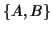and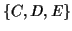are grouped together. But at the same time sequencesand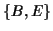should also be grouped together, due to event 4, which leads to a contradiction. Generally we define a conflict as follows:

Definition 4.1 (Conflict)   Given an MSA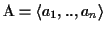and two indel events (gap blocks) that split the sequences into the sets A1, B1 (event 1) and A2, B2 (event 2). Then event 1 and event 2 have a conflict, if,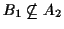,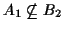and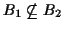(see Figure).

Finding the minimum number of events to ignore such that we have no conflicts is called the "vertex cover problem" in graph theory.

Problem 4.1 (Vertex Cover)   Given is a graph G = (V, E) with vertices V and edges E. The problem is to find the smallest subset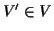such that for each edge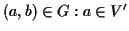or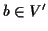.

In our case each indel event (or gap block) is a vertex, and whenever there is a conflict between two events an edge is drawn that connects the two vertices. A minimum vertex cover corresponds to a minimum number of events such that the MSA ignoring those events is conflict free. Once all conflicts are removed, we can build an evolutionary tree from the remaining events (if we have enough events).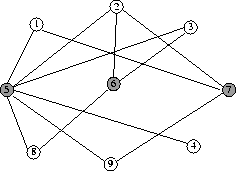The problem of finding the minimum vertex cover is NP-complete . But usually the number of indel events is small (smaller than 50), when the size of the MSA is restricted to the size of a domain (which is usually at most 70 amino acids long). Vertex cover problems of this magnitude can be readily solved, there is a fixed parameter tractable algorithm  to solve the problem deterministically, when parameterized by the size of the vertex cover [26,29]. With this algorithm, conflict graphs with minimum vertex covers of size larger than 150 can be resolved in reasonable time. A more detailed version of the paper can be obtained at
http://www.inf.ethz.ch/personal/korosten/papers/gaps.ps. All algorithms described here have been implemented into the Darwin system  and are available upon request. The programs can also be used via our computational biochemistry server: http://cbrg.ethz.ch.Next: Bibliography Up: Tree construction using gaps Previous: Tree construction using gaps
Chantal Korostensky
1999-07-14